SYMMICTM Users Manual
Version 3.1.4

## Choosing Appropriate Boundary Conditions

The principal method of device cooling in most templates is through a heat transfer (a.k.a. film) boundary condition applied to the bottom (backside) of the device or layout. Templates with cooling through the sides or other surfaces are possible but require careful consideration of the computational method being used. Most importantly, when solving a layout it is assumed that every device in the layout is subject to the same backside boundary conditions and that these boundary conditions are properly flagged as belonging to the layout in every device template, (see Device Template File Format in the Appendices for details.) Attempting to apply different values for Backside Film Temperature and Backside Film Coefficient in different devices in a layout may not create a well-defined problem from the point of view of superposition and therefore may not produce an accurate result. However, it is possible to create a differential distribution of temperatures on the bottom of a layout by applying a boundary condition from a file. In this case every device simulation uses the same temperature distribution, whereas in the case of inconsistent film parameters, each device is specifying a different backside temperature for the layout. In general, it is best to make sure that the backside film parameters are equalized over the layout so that the boundary conditions are always consistent.

All units in SYMMIC are standard international units referenced to micrometers (a.k.a. microns), so film boundary conditions are expected in W/µm2 °K for the heat transfer coefficient, and in Kelvin for the environmental temperature. As noted above, in this system of units a heat transfer coefficient of 1 is a very large (unrealistic) value. More realistic heat transfer coefficient ranges are given in the table below.

Magnitude Range of Heat Transfer Coefficients

 Surface Cooling Method BTU/h ft2 °F W/m2 °K W/µm2 °K Natural convection of gases* 0.9 to 5 5 to 30 5e-12 to 3e-11 Laminar flowing gases* 2 to 50 10 to 300 1e-11 to 3e-10 Turbulent gas flows (100-2,000 m/s) 100 to 1,000 500 to 5,000 5e-10 to 5e-9 Flowing non-metallic liquids* 30 to 1,000 200 to 6,000 2e-10 to 6e-9 Microjet air impingement§ 200 to 3,600 1,000 to 20,000 1e-9 to 2e-8 Condensing vapors* 500 to 5,000 3,000 to 30,000 3e-9 to 3e-8 Flowing liquid metals* 1,000 to 50,000 6,000 to 300,000 6e-9 to 3e-7 Boiling liquids* 200 to 50,000 1,000 to 300,000 1e-9 to 3e-7 Microjet water impingement§ 10,000 to 100,000 50,000 to 500,000 5e-8 to 5e-7 Microjet boiling impingement§ 0.4 to 2 million 2 to 10 million 2e-6 to 1e-5

*Rohsennow M. W., Choi H. (1961) Heat, Mass, and Momentum Transfer. Prentice-Hall, Inc. Englewood Cliff, NJ, p. 102.

§ CapeSym, Inc. measurement data

Assuming the surface area available for cooling was equivalent to the backside area of the device or layout, heat transfer coefficients taken from this table would give a reasonable estimate of thermal performance of the real system provided the environmental temperature is also known. Other factors affecting the heat transfer coefficient are the rate and type of flow (laminar versus turbulent) across the surface, and the nature of the convective medium (gaseous versus liquid).

To aid in the calculation of the heat transfer coefficient, some templates may provide a calculator. Details on using the calculator for various types of heat sinks are given in the next section.

Please note: To use the Heat Transfer Coefficient Calculator your device template must have the calculator option enabled and it must contain one or more fluid materials.

### Heat Transfer Coefficient Calculator

SYMMIC has the capability to calculate heat transfer coefficient values for many different kinds of convective phenomena, so that the user can quickly check the device temperatures with different cooling strategies. The calculator can also be used to input the thermal resistance value of a certain heat sink configuration, which manufacturers typically provide, to see how well that heat sink would cool the device.

This calculator is accessed by the Calculate button on the dialog box for selected parameters. A parameter only has this button enabled if the template has it linked in (link="HTCOEFF", see Device Template File Format in the Appendices for details). In addition, the desired cooling fluid needs to be a material in the template. A material is considered a fluid if it has the viscosity property defined. For the Generic FET Template, the heat transfer coefficient calculator is enabled for the Backside Film Coefficient, and the fluid materials Air, Water, and FC-77 are included.

The heat transfer coefficient calculator dialog is the same for every heat transfer type, but different entries are needed for each. So the user should begin by selecting the Heat Transfer Type from the pull-down list of options. Directly below this selection are one to three dimensions to be specified, and in the case of “Thermal Resistance,” the resistance value. Below the geometry definition is a pull-down list to select the “Heat Transfer Fluid.” Finishing out the left side of the dialog are the Flow Velocity and Flow Temp specifications.

On the right side of the dialog are the fin specification fields, which are only active for a “Finned Heat Sink” type. The Fin Type can be either “Straight Rectangular,” as for a typical extruded heat sink, “Aligned Pin Fin Array,” or “Staggered Pin Fin Array.” Below the Fin Type selection are the fin dimensions, followed by a Fin Material selection. The remaining specifications are for the array, which is constrained to only 1 Rows for the extruded fin type, but can have multiple Rows and Cols (columns) for the pin fin array types. The Crossflow Spacing is the distance between Cols, and the Streamwise Spacing is the distance between Rows. At the bottom is a brief instructional message, a display of the current value for the Calculated Heat Transfer Coefficient, and the OK and Cancel buttons. If OK is pressed, then the coefficient value displayed is applied to the associated boundary condition parameter.

The heat transfer coefficient calculations are based on established empirical formulas which relate geometrical and fluid parameters to the rate of heat transfer between the fluid and the solid. Most of these formulas were developed from experiments with common fluids such as water and air. If other fluids are used, particularly those with radically different Prandtl numbers (like liquid metals), then some of the formulas will try and compensate, but not all. In general, for exotic fluids one should expect the formulas to be less accurate. The following section will describe each heat transfer type, what is required from the user, and how the coefficient is calculated.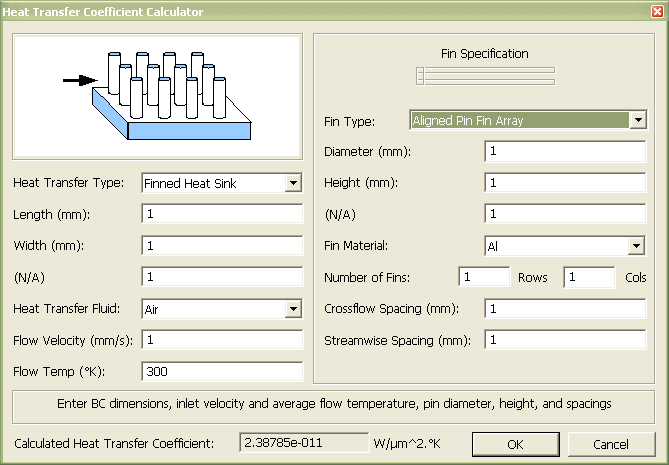### Heat Transfer Types

The heat transfer types available in the heat transfer coefficient calculator are thermal resistance (for input of a manufacturer's heat sink information) and forced convection. The forced convection types are: flow over a flat plate, between parallel plates, in a channel, or in a pipe; impingement flow (i.e. normal flow of a submerged jet); and flow through a finned heat sink, where the fins can be straight (rectangular), aligned pins, or staggered pins.

All heat transfer types, except for thermal resistance, require the input of an average flow temperature. Note that this same temperature value must be entered for the heat sink temperature used by the heat transfer coefficient being calculated, which in the case of the Generic FET Template, is called the Backside Film Temperature.

Thermal Resistance. Enter the heat sink thermal resistance (Rth) and the dimensions of the BC area, length (L) by width (W).

Heat sinks are often specified in terms of an effective thermal resistance for a given air flow rate. For example, at the time of this writing AAVID THERMALLOY provides resistance calculations for its heat sinks online at: http://www.aavidthermalloy.com/products/extrusion/products.shtml

The “BC area” dimensions refers to the area over which this boundary condition will be applied, which may be different from the dimensions of the heat sink. For the Backside Film Coefficient of the Generic FET Template, it is the entire backside area of the FET. The current dimensions of the BC area are available in the “Derived Parameters” group (be sure to convert from microns to millimeters).

The thermal resistance can be converted to the heat transfer coefficient (h) by multiplying by the BC area and inverting the result: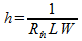Note that the next five heat transfer types do not relate as directly to the BC geometry of the template as L and W in this type did.

Flat Plate. Enter streamwise length (L) of the plate, the flow velocity (V) and average temperature (T).

This heat transfer coefficient assumes a 2D flat plate flow, with infinite (cross-stream) width and a given length. The flow velocity specification is the free stream value (far from the plate); the correlation relates the velocity and the streamwise length to the development of the boundary layer thickness. The flow temperature value should be the average of the free stream and the (expected) flat plate surface temperatures. As noted above, don't forget to enter this same temperature as the heat sink temperature used by the heat transfer coefficient being calculated, which in the case of the Generic FET Template, is called the Backside Film Temperature.

To make the calculation, the Reynolds (ReL), Prandtl (Pr) and Nusselt (NuL) numbers (based on length, L) are needed, and they use the fluid properties at the given temperature.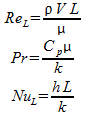The fluid properties are density (ρ), viscosity (μ), specific heat (Cp), and thermal conductivity (k).

The Reynolds number is used to determine how much of the flat plate boundary layer is turbulent; a transition Retr of 250,000 is currently used (it can range from 50,000 to 500,000 in practice). If the flow is laminar, then the correlation used depends on the Prandtl number, as follows from Mills, equations (4.57) and (4.58).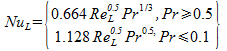If Pr is in between 0.1 and 0.5, then linear interpolation is used.

If ReL is greater than Retr, then the turbulent correlation is used for that portion of the plate length that is turbulent. Only the high-Prandtl-number version is known, from Mills equation (4.65).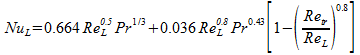Once NuL is known, then h can be calculated using the Nusselt number definition above. (The turbulent correlation above is only recommended for ReL < 3 x 107.)

Pipe Flow. Enter the streamwise length (L) of the pipe, its diameter (D), the flow velocity (V) and average temperature (T).

Flow in a straight pipe has often been studied and the correlations for it are well established. The applicable Reynolds and Nusselt numbers are based on the pipe diameter, while the pipe length is compared to the development length of the boundary layer.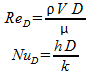The transitional Reynolds number for pipes (Retr) is 2300. If ReD is less than Retr, then the flow is laminar, and described by the Mills equation (4.50).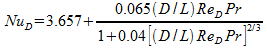Transitional flow are those which are neither fully turbulent nor fully laminar. The Kays and London correlation (from Fig. 7-3, 7-4) applies to pipe flow with Retr < ReD < 6000.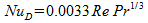For fully turbulent flows, correlations exist for both high and low Prandtl numbers, from Mills equations (4.42), (4.45) and (4.46).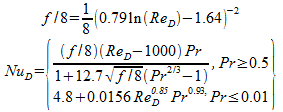As before, if the Prandtl number falls between 0.5 and 0.01, linear interpolation is used. Also, the turbulent correlation above is only recommended for ReD < 1 x 106. Finally, these correlations assume a constant axial wall temperature.

Parallel Flat Plates. Enter the streamwise length (L) of the plates, their spacing (H), the flow velocity (V) and average temperature (T).

The heat transfer coefficient for a pair of flat plates uses the same correlations as those for pipe flow, but with an equivalent diameter dimension (a.k.a. “hydraulic diameter”).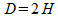Channel Flow. Enter the streamwise length (L) of the channel, its height (H) and width (W), the flow velocity (V) and average temperature (T).

A channel with rectangular cross-section is similar to a channel with circular cross-section (i.e. a pipe). As for Parallel Flat Plates, the first step is to define the hydraulic diameter.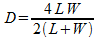Since the Nusselt correlation varies with the aspect ratio of the channel, the following factor is defined based on Mills Table 4.5.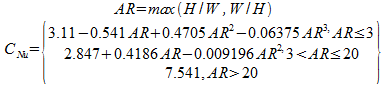The transitional Retr is defined as 2300, as for pipe flow. Then, for laminar flow ( Retr < 2300), a variant of the Mills equation (4.50) applies.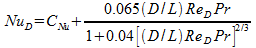For larger Reynolds numbers, the flow transitions to turbulent and the CNu factor no longer applies. The Pipe Flow correlations are applied directly.

Impingement. Enter the impingement nozzle diameter (Dn), its spacing from the surface (S), the surface dimension (L), the flow velocity (V) and average temperature (T).

In impingement, or normal flow, a jet of fluid impacts a surface and creates a boundary layer as it flows out radially. These calculations are for submerged jets, where the fluid of the jet is the same as the surrounding fluid (e.g. not a water jet on a surface surrounded by air).

These correlations were developed for air, water and FC-77; their validity with dissimilar fluids is unknown. Different correlations are used for gases and liquids in SYMMIC, by simply comparing the fluid density to a value of 50 kg/m3 (if less, then the gas correlation is used, otherwise the liquid correlation is used).

The jet Reynolds number, ReDn, is based on the nozzle diameter. The surface dimension, L, assumes that the BC area (L2) is a square region. It is converted to an equivalent diameter dimension, Dequiv, by the following from Incropera, equation (5.63).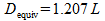For gases, the correlation used is from Incropera, equation (5.62).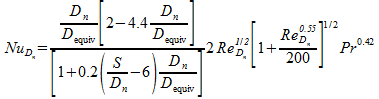The parameters above are limited to the correlation ranges before calculation (i.e. these ranges are enforced): 2 < S / Dn < 12, 5 < Dequiv / Dn < 15. The Reynolds number range of the correlation is 2000 < ReDn < 4 x 105, and the Prandtl range is unknown; these are not enforced in the calculation.

Because heat transfer from an impingement surface is caused by a variety of different boundary layer regions (a stagnation zone under the jet, progressing to a laminar region and then transition to turbulence at a certain radial distance), the liquid jet correlations used differ with the relative size of the impingement surface. The average length of the wall jet region (stagnation and laminar flow), L*, is described by Incropera, equation (5.61).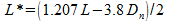The non-dimensional area of the wall jet region is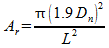If the wall jet area is less than 1 (i.e. no turbulent region), Ar < 1, then (Incropera, equation (5.60))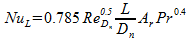Otherwise, the full equation (5.60) is used.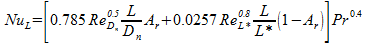This equation is valid for the parameter ranges 1.9 < L / Dn < 13 (enforced) as well as 1 < S / Dn < 4, 0.978 < Dn < 6.55 mm and L = 12.7 mm (not enforced).

Incropera also gives another correlation for submerged liquid jets, equation (5.59).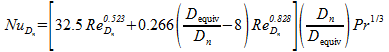This equation is valid for the parameter ranges 8 < Dequiv / Dn < 58 (enforced), as well as 1.74 < Dn < 12.7 and 1 < S / Dn < 7 (not enforced). The Reynolds number range tested was 2000 < ReDn < 40000.

SYMMIC reports the average of these two correlations for an impinging liquid jet.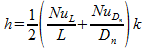Finned Heat Sink. All of the finned heat sink types ask for the BC dimensions, length (L) and width (W), the inlet flow velocity (V) and average temperature (T). The average temperature of the inlet, free stream flow and the heat sink surface is as before, but the flow velocity specification is at the inlet, before the flow is disturbed by the fins. The BC dimensions are required in addition to the heat sink dimensions because the two may be different and the final heat transfer coefficient is for the BC surface. (Note that no spreading resistance is calculated, and for large dimensional disparities it may be non-negligible.) The two BC dimensions, which should be available in the “Derived Parameters” list, are simply multiplied to get the BC area (A).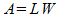Finned Heat Sink, Straight Rectangular. Enter the BC dimensions, inlet flow velocity (V) and average temperature (T), fin thickness (Df), height (Wf) and streamwise length (Lf).

The fin heat transfer coefficient, hf, is found using the Flat Plate correlations above, based on the streamwise length Lf of the fins (in place of the flat plate length L).

Once the fin heat transfer coefficient is known, then the fin efficiency, n, can be calculated (Mills, Table 2.2), which accounts for the temperature gradient in the fin from the base (used for the average temperature input) to the tip.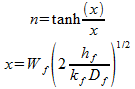where kf is the thermal conductivity of the fin material.

With the fin efficiency known, the heat transfer coefficient for the BC area can be calculated.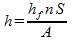where S is the fin area. If Nc is the number of fins (“ncols”), then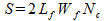Finned Heat Sink, Aligned Pin Fin Array. Enter the BC dimensions, inlet flow velocity (V) and average temperature (T), pin diameter (Df), height (Wf) and spacings: number of rows (Nr), number of columns (Nc), crossflow spacing (ST), and streamwise spacing (SL).

The fin heat transfer coefficient, hf, for pin fin arrays uses the correlations for flow through tube banks. The average velocity between the pins, Vf, is given by Mills equation (4.113).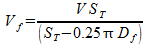The Reynolds number, Re, is based on this velocity.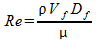The Nusselt number for an individual pin, NuD1, comes from correlations for flow over a cylinder (Kreith Table 9-1, Mills equation (4.71)). For low Reynolds numbers, Re < 40: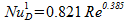For Re < 104: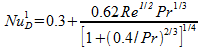For 104 < Re < 4 x 105: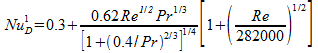Finally, for the largest Re (suggested 4 x 105 < Re < 5 x 106):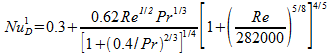The two spacings, ST and SL, are from pin center to center, and pins must not overlap, so both must be greater than the pin diameter, Df. Given that requirement, dimensionless crossflow (transverse) and streamwise (longitudinal) pitch is defined by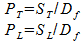And from those, a factor ψ can be defined by Mills, equation (4.115).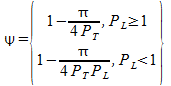For an aligned pin array, the arrangement factor, φA, is defined by Mills, equation (4.116).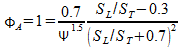If the array contains at least 10 rows, Nr >= 10, then the array Nusselt number is defined by Mills, equation (4.114).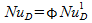where φ = φA for an aligned array, and φ = φS for a staggered array. For less than 10 rows, linear interpolation applies (equation (4.118)).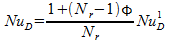Finally, the fin heat transfer coefficient, hf, is calculated from NuD. Once the fin heat transfer coefficient is known, then the fin efficiency, n, can be calculated (Mills, Table 2.2), which for pin fin arrays is as follows.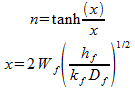where kf is the thermal conductivity of the fin material.

With the fin efficiency known, the heat transfer coefficient for the BC area can be calculated.where S is the fin area. If NrNc is the number of fins, then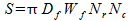Finned Heat Sink, Staggered Pin Fin Array. Enter the BC dimensions, inlet flow velocity (V) and average temperature (T), pin diameter (Df), height (Wf) and spacings: number of rows (Nr), number of columns (Nc), crossflow spacing (ST), and streamwise spacing (SL).

The staggered pin fin array is treated the same as the aligned array, except for the arrangement factor. For this equation, there is disagreement in various editions of Mills, so SYMMIC uses the following adaptation of equation (4.117).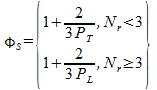Summary. In each case, once the desired entries have been made, to apply the calculated heat transfer coefficient press the OK button. Then select the associated sink temperature parameter (T), which for the Generic FET Template is called the Backside Film Temperature, and enter the average flow temperature value used for the heat transfer coefficient calculation (or in the case of Thermal Resistance, enter the desired average flow temperature).

### References Cited

1. Basic Heat and Mass Transfer, Mills, A. F.; Richard D. Irwin, Inc.,1995.

2. Compact Heat Exchangers, 3rd edition, Kays, W. M., and London, A. L.; Krieger Publishing Company, 1998.

3. Liquid Cooling of Electronic Devices by Single-Phase Convection, Incropera, F. P., John Wiley & Sons, Inc., 1999.

4. Principles of Heat Transfer, 3rd edition, Kreith, F.; Harper and Row Publishers, Inc., 1973.